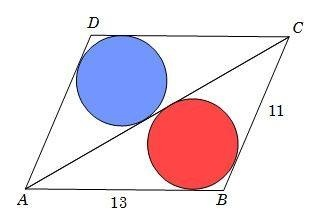# A Parallelogram and 2 Circles

Geometry Level 4In Parallelogram ABCD, $AB=13$, $BC=11$, and $AC=20$. Two congruent circles are inscribed in $\triangle ACD$ and $\triangle ACB$ as shown in the figure.

The shortest distance between the centers of the two inscribed circles can be expressed in the form $a\sqrt{b}$. Where $b$ is a square-free integer. Find the value of $a+b$.

• Found this somewhere.
×